Do you like the article?
Share it with others -
post a link to it!
Use new possibilities of MetaTrader 5

# Advanced Adaptive Indicators Theory and Implementation in MQL5

31 May 2011, 18:00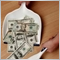9
20 758

### Introduction

This article is based on two excellent books by John F. Ehlers: "Rocket Science for Traders" and "Сybernetic Analysis for Stock and Futures". The unusual approach to market analysis using digital signal processing methods and adopting complex numbers to market cycle recognition made me go deeper into this subject and subsequently implement in MQL5 three adaptive indicators presented by J.F.Ehlers.

This article will describe the basic theory behind the adaptive indicators and their MQL5 implementation. The adaptive indicators will be compared to their non-adaptive counterparts.

### Complex numbers and phasors for measuring market cycles

The notion of complex numbers theory may be quite perplexing for readers having non-engineering background, therefore I recommend to delve into theory on wiki and watch a tutorial on operations on complex numbers before reading this article.

Phasor

Phasor or Phase Vector is a vector that shows amplitude and phase of a cycle. According to Euler's formula a sinewave can be represented as a sum of two complex number components. Please observe rotating phasor depicting a sinewave cycle below.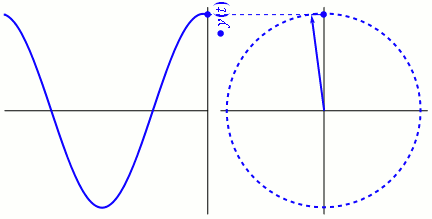Seeing this animation for the first time you may be puzzled how to correctly read phasor relation to a cycle. In order to understand that you have to switch your mind to recognize a cycle not as a usual waveform visible on the left part of the animation, but as the rotating phasor on the right.

At first this may be hard to imagine, but I found a way to think about it this way: full rotation of a phasor is 360 degrees or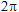radian, the same is for a full cycle. The current angle of a phasor indicates in which part of the cycle (phase) we are in. Y axis represents amplitude of a cycle in a given phase.

The phasor can be broken into two components: InPhase component (cosine) and Quadrature component (sine). Detailed explanation of deriving those components is available in Chapter 6 "Hilbert Transforms" of "Rocket Science for Traders" book. If anyone is interested, please follow this chapter carefully.

For now you only need to concentrate on the fact that for adaptive indicators calculation we need to convert analytic signal (waveform) to a complex signal composed of two components. How do we achieve that? Did I mention Hilbert Transform? Yes, indeed. Hilbert Transform is capable of just that.

### Measuring cycle period

In order to make Hilbert Transform practical to traders John Ehlers in his book truncated Hilbert Transform series to four elements.

The equation for Quadrature component is: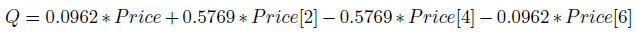and the equation for InPhase component is price delayed by three bars: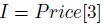Having calculated InPhase and Quadrature components it is possible to derive differential phase calculation from the phase angle measured for the current bar and the phase angle measured one bar ago. Phase for the current bar is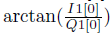and phase for the previous bar is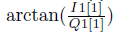. Using trigonometric identity: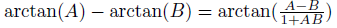we obtain equation for differential phase reffered to as DeltaPhase.

Mr. Ehlers put additional constraints on the DeltaPhase variable: the result cannot be negative and DeltaPhase is restricted to <0.1, 1.1> radians (meaning a cycle between 6 and 63 bars). It appeared that DeltaPhase measured on real data is very noisy, therefore it needs smoothing.

The best smoothing method on spiky data is median filter, therefore a median of five samples of DeltaPhase forms MedianDelta variable. MedianDelta divided byis used for computing the Dominant Cycle, the market cycle we are looking for.

During development tests it turned out that there is a bias of about 0.5 in measurement that needs to be removed and compensation term to remove that bias was added. Finally, the Dominant Cycle is smoothed twice by EMA with alpha values equal to 0.33 and 0.15, respectively. I really recommend to read the book to see robustness of the algorithm applied to a sinewave whose cycle period gradually increased from 6 to 40.

Since you are equipped with theoretical knowledge we are ready to implement the CyclePeriod indicator in MQL5.

### Cycle Period indicator

The indicator is composed of two lines: cycle line showing cycle period and a trigger line, which basically is a cycle line delayed by one bar. If you follow the description in "Measuring cycle period" section and the source code in OnCalculate() function you will be easily able to correlate which lines are responsible for cycle period measurement.

```//+------------------------------------------------------------------+
//|                                                  CyclePeriod.mq5 |
//|                                      Copyright 2011, Investeo.pl |
//|                                               http://Investeo.pl |
//+------------------------------------------------------------------+
#property copyright "Copyright 2011, Investeo.pl"
#property link      "http://Investeo.pl"
#property version   "1.00"
#property indicator_separate_window

#property description "CyclePeriod indicator - described by John F. Ehlers"
#property description "in \"Cybernetic Analysis for Stocks and Futures\""

#property indicator_buffers 2
#property indicator_plots 2
#property indicator_width1 1
#property indicator_width2 1
#property indicator_type1   DRAW_LINE
#property indicator_type2   DRAW_LINE
#property indicator_color1  Green
#property indicator_color2  Red
#property indicator_label1  "Cycle"
#property indicator_label2  "Trigger Line"

#define Price(i) ((high[i]+low[i])/2.0)

double Smooth[];
double Cycle[];
double Trigger[];
//double Price[];
double Q1[]; // Quadrature component
double I1[]; // InPhase component
double DeltaPhase[];
double InstPeriod[];
double CyclePeriod[];

input double InpAlpha=0.07; // alpha
//+------------------------------------------------------------------+
//| Custom indicator initialization function                         |
//+------------------------------------------------------------------+
int OnInit()
{
//--- indicator buffers mapping
ArraySetAsSeries(Cycle,true);
ArraySetAsSeries(CyclePeriod,true);
ArraySetAsSeries(Trigger,true);
ArraySetAsSeries(Smooth,true);
//ArraySetAsSeries(Price,true);

SetIndexBuffer(0,CyclePeriod,INDICATOR_DATA);
SetIndexBuffer(1,Trigger,INDICATOR_DATA);

PlotIndexSetDouble(0,PLOT_EMPTY_VALUE,0.0);
PlotIndexSetDouble(1,PLOT_EMPTY_VALUE,0.0);

return(0);
}
//+------------------------------------------------------------------+
//| Custom indicator iteration function                              |
//+------------------------------------------------------------------+
int OnCalculate(const int rates_total,
const int prev_calculated,
const datetime &time[],
const double &open[],
const double &high[],
const double &low[],
const double &close[],
const long &tick_volume[],
const long &volume[],
const int &spread[])
{
//---
long tickCnt;
int i;
int ticks=CopyTickVolume(Symbol(), 0, 0, 1, tickCnt);
if(ticks!=1) return(rates_total);
double DC, MedianDelta;

Comment(tickCnt);

if(prev_calculated==0 || tickCnt==1)
{
//--- last counted bar will be recounted
int nLimit=rates_total-prev_calculated-1; // start index for calculations

ArraySetAsSeries(high,true);
ArraySetAsSeries(low,true);

ArrayResize(Smooth,Bars(_Symbol,_Period));
ArrayResize(Cycle,Bars(_Symbol,_Period));
//ArrayResize(Price,Bars(_Symbol,_Period));
ArrayResize(CyclePeriod,Bars(_Symbol,_Period));
ArrayResize(InstPeriod,Bars(_Symbol,_Period));
ArrayResize(Q1,Bars(_Symbol,_Period));
ArrayResize(I1,Bars(_Symbol,_Period));
ArrayResize(DeltaPhase,Bars(_Symbol,_Period));

if (nLimit>rates_total-7) // adjust for last bars
nLimit=rates_total-7;

for(i=nLimit;i>=0 && !IsStopped();i--)
{
Smooth[i] = (Price(i)+2*Price(i+1)+2*Price(i+2)+Price(i+3))/6.0;

if (i<rates_total-7)
{
Cycle[i] = (1.0-0.5*InpAlpha) * (1.0-0.5*InpAlpha) * (Smooth[i]-2.0*Smooth[i+1]+Smooth[i+2])
+2.0*(1.0-InpAlpha)*Cycle[i+1]-(1.0-InpAlpha)*(1.0-InpAlpha)*Cycle[i+2];

} else
{
Cycle[i]=(Price(i)-2.0*Price(i+1)+Price(i+2))/4.0;
}

Q1[i] = (0.0962*Cycle[i]+0.5769*Cycle[i+2]-0.5769*Cycle[i+4]-0.0962*Cycle[i+6])*(0.5+0.08*InstPeriod[i+1]);
I1[i] = Cycle[i+3];

if (Q1[i]!=0.0 && Q1[i+1]!=0.0)
DeltaPhase[i] = (I1[i]/Q1[i]-I1[i+1]/Q1[i+1])/(1.0+I1[i]*I1[i+1]/(Q1[i]*Q1[i+1]));
if (DeltaPhase[i] < 0.1)
DeltaPhase[i] = 0.1;
if (DeltaPhase[i] > 0.9)
DeltaPhase[i] = 0.9;

MedianDelta = Median(DeltaPhase, i, 5);

if (MedianDelta == 0.0)
DC = 15.0;
else
DC = (6.28318/MedianDelta) + 0.5;

InstPeriod[i] = 0.33 * DC + 0.67 * InstPeriod[i+1];
CyclePeriod[i] = 0.15 * InstPeriod[i] + 0.85 * CyclePeriod[i+1];
Trigger[i] = CyclePeriod[i+1];
}
}
//--- return value of prev_calculated for next call
return(rates_total);
}
//+------------------------------------------------------------------+

double Median(double& arr[], int idx, int m_len)
{
double MedianArr[];
int copied;
double result = 0.0;

ArraySetAsSeries(MedianArr, true);
ArrayResize(MedianArr, m_len);

copied = ArrayCopy(MedianArr, arr, 0, idx, m_len);
if (copied == m_len)
{
ArraySort(MedianArr);
if (m_len %2 == 0)
result = (MedianArr[m_len/2] + MedianArr[(m_len/2)+1])/2.0;
else
result = MedianArr[m_len / 2];

}
else Print(__FILE__+__FUNCTION__+"median error - wrong number of elements copied.");
return result;
}```

We can test it by attaching to any chart - it will work for any security and for any timeframe.

Please see the screenshot below.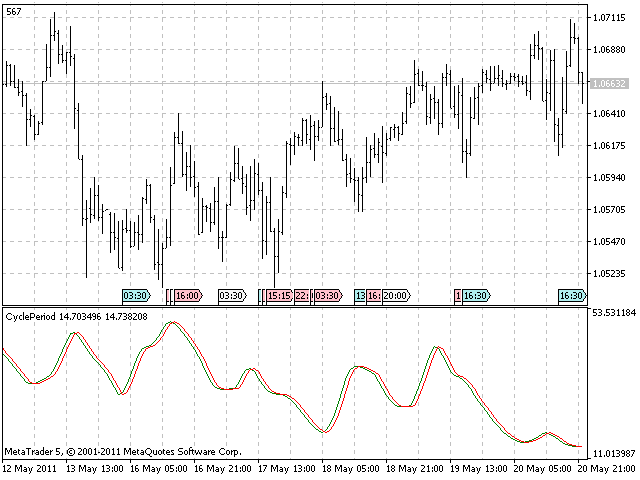With this indicator in our workspace we are able to implement a new breed of adaptive indicators - indicators that adapt to a current cycle period of the market.

### Cyber Cycle indicator

The Cyber Cycle indicator is a high-pass filter taken from "Сybernetic analysis for stocks and futures". This filter leaves only the cycle mode component from timeseries.

Additionaly two-bar and three-bar cycle components are extracted from the result by smoothing it with a finite impulse response low pass filter.

The indicator MQL5 code for this and other indicators in the article adapted from EFL (Tradestation) language described in the book.

```//+------------------------------------------------------------------+
//|                                                   CyberCycle.mq5 |
//|                                      Copyright 2011, Investeo.pl |
//|                                               http://Investeo.pl |
//+------------------------------------------------------------------+
#property copyright "Copyright 2011, Investeo.pl"
#property link      "http://Investeo.pl"
#property version   "1.00"
#property indicator_separate_window

#property description "CyberCycle indicator - described by John F. Ehlers"
#property description "in \"Cybernetic Analysis for Stocks and Futures\""
#property description "This indicator is available for free download."

#property indicator_buffers 2
#property indicator_plots 2
#property indicator_width1 1
#property indicator_width2 1
#property indicator_type1   DRAW_LINE
#property indicator_type2   DRAW_LINE
#property indicator_color1  Green
#property indicator_color2  Red
#property indicator_label1  "Cycle"
#property indicator_label2  "Trigger Line"

#define Price(i) ((high[i]+low[i])/2.0)

double Smooth[];
double Cycle[];
double Trigger[];

input double InpAlpha=0.07; // alpha
//+------------------------------------------------------------------+
//| Custom indicator initialization function                         |
//+------------------------------------------------------------------+
int OnInit()
{
//--- indicator buffers mapping
ArraySetAsSeries(Cycle,true);
ArraySetAsSeries(Trigger,true);
ArraySetAsSeries(Smooth,true);

SetIndexBuffer(0,Cycle,INDICATOR_DATA);
SetIndexBuffer(1,Trigger,INDICATOR_DATA);

PlotIndexSetDouble(0,PLOT_EMPTY_VALUE,0.0);
PlotIndexSetDouble(1,PLOT_EMPTY_VALUE,0.0);

return(0);
}
//+------------------------------------------------------------------+
//| Custom indicator iteration function                              |
//+------------------------------------------------------------------+
int OnCalculate(const int rates_total,
const int prev_calculated,
const datetime &time[],
const double &open[],
const double &high[],
const double &low[],
const double &close[],
const long &tick_volume[],
const long &volume[],
const int &spread[])
{
//---
long tickCnt;
int i;
int ticks=CopyTickVolume(Symbol(), 0, 0, 1, tickCnt);
if(ticks!=1) return(rates_total);

Comment(tickCnt);

if(prev_calculated==0 || tickCnt==1)
{
//--- last counted bar will be recounted
int nLimit=rates_total-prev_calculated-1; // start index for calculations

ArraySetAsSeries(high,true);
ArraySetAsSeries(low,true);

ArrayResize(Smooth,Bars(_Symbol,_Period));
ArrayResize(Cycle,Bars(_Symbol,_Period));

if(nLimit>rates_total-4) // adjust for last bars
nLimit=rates_total-4;

for(i=nLimit;i>=0 && !IsStopped();i--)
{
Smooth[i]=(Price(i)+2*Price(i+1)+2*Price(i+2)+Price(i+3))/6.0;

if(i<rates_total-5)
{
Cycle[i]=(1.0-0.5*InpAlpha) *(1.0-0.5*InpAlpha) *(Smooth[i]-2.0*Smooth[i+1]+Smooth[i+2])
+2.0*(1.0-InpAlpha)*Cycle[i+1]-(1.0-InpAlpha)*(1.0-InpAlpha)*Cycle[i+2];
}
else
{
Cycle[i]=(Price(i)-2.0*Price(i+1)+Price(i+2))/4.0;
}

//Print(__FILE__+__FUNCTION__+" received values: ",rCnt);
Trigger[i]=Cycle[i+1];
}
}
//--- return value of prev_calculated for next call
return(rates_total);
}
//+------------------------------------------------------------------+
```

A screenshot of the indicator is pasted below.

As you may notice all indicators in this article will have similar look, but they implement very different algorithms.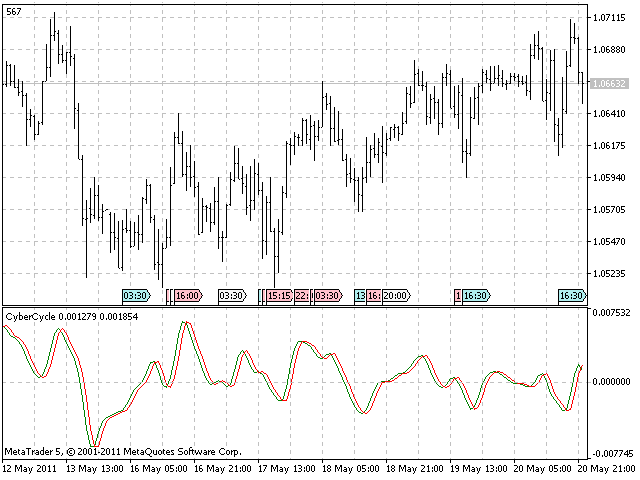The original trading method for this indicator is straightforward: buy when the cycle line crosses above the trigger line. Sell when the cycle line crosses under the trigger line. You may want to and you are encouraged to implement your own strategy and a module of trading signals using this indicator.

### Adaptive Cyber Cycle indicator

The essence of this article is to present how can we make the indicators to be adaptive, that is how to calculate them with dynamic cycle period inputs instead of a static setting. In order to achieve that, we have to connect to the CyclePeriod indicator to read the current period and later use this reading in OnCalculate() function.

At first we need to get the indicator's handle:

```hCyclePeriod=iCustom(NULL,0,"CyclePeriod",InpAlpha);
if(hCyclePeriod==INVALID_HANDLE)
{
Print("CyclePeriod indicator not available!");
return(-1);
}```

and then read it inside OnCalculate() function:

```int copied=CopyBuffer(hCyclePeriod,0,i,1,CyclePeriod);
if(copied<=0)
{
Print("FAILURE: Could not get values from CyclePeriod indicator.");
return -1;
}
alpha1 = 2.0/(CyclePeriod+1.0);```

Expotential moving alpha is related to the length of a simple moving average by the equation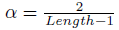, in the Adaptive Cyber Cycle indicator Mr. Ehlers used the Dominant Cycle period as the length in computation of alpha1 coefficient.

Full source code is available below:

```//+------------------------------------------------------------------+
//|                                           AdaptiveCyberCycle.mq5 |
//|                                      Copyright 2011, Investeo.pl |
//|                                               http://Investeo.pl |
//+------------------------------------------------------------------+
#property copyright "Copyright 2011, Investeo.pl"
#property link      "http://Investeo.pl"
#property version   "1.00"
#property indicator_separate_window

#property description "Adaptive CyberCycle indicator - described by John F. Ehlers"
#property description "in \"Cybernetic Analysis for Stocks and Futures\""
#property description "This indicator is available for free download."

#property indicator_buffers 2
#property indicator_plots 2
#property indicator_width1 1
#property indicator_width2 1
#property indicator_type1   DRAW_LINE
#property indicator_type2   DRAW_LINE
#property indicator_color1  Green
#property indicator_color2  Red
#property indicator_label1  "Cycle"
#property indicator_label2  "Trigger Line"

#define Price(i) ((high[i]+low[i])/2.0)

double Smooth[];
double Cycle[];
double Trigger[];

int hCyclePeriod;

input double InpAlpha=0.07; // alpha for Cycle Period
//+------------------------------------------------------------------+
//| Custom indicator initialization function                         |
//+------------------------------------------------------------------+
int OnInit()
{
//--- indicator buffers mapping
ArraySetAsSeries(Cycle,true);
ArraySetAsSeries(Trigger,true);
ArraySetAsSeries(Smooth,true);

SetIndexBuffer(0,Cycle,INDICATOR_DATA);
SetIndexBuffer(1,Trigger,INDICATOR_DATA);

PlotIndexSetDouble(0,PLOT_EMPTY_VALUE,0.0);
PlotIndexSetDouble(1,PLOT_EMPTY_VALUE,0.0);

hCyclePeriod=iCustom(NULL,0,"CyclePeriod",InpAlpha);
if(hCyclePeriod==INVALID_HANDLE)
{
Print("CyclePeriod indicator not available!");
return(-1);
}
return(0);
}
//+------------------------------------------------------------------+
//| Custom indicator iteration function                              |
//+------------------------------------------------------------------+
int OnCalculate(const int rates_total,
const int prev_calculated,
const datetime &time[],
const double &open[],
const double &high[],
const double &low[],
const double &close[],
const long &tick_volume[],
const long &volume[],
const int &spread[])
{
//---
long tickCnt;
int i;
int ticks=CopyTickVolume(Symbol(), 0, 0, 1, tickCnt);
if(ticks!=1) return(rates_total);
double CyclePeriod,alpha1;

Comment(tickCnt);

if(prev_calculated==0 || tickCnt==1)
{
//--- last counted bar will be recounted
int nLimit=rates_total-prev_calculated-1; // start index for calculations

ArraySetAsSeries(high,true);
ArraySetAsSeries(low,true);

ArrayResize(Smooth,Bars(_Symbol,_Period));
ArrayResize(Cycle,Bars(_Symbol,_Period));

if(nLimit>rates_total-4) // adjust for last bars
nLimit=rates_total-4;

for(i=nLimit;i>=0 && !IsStopped();i--)
{
Smooth[i]=(Price(i)+2*Price(i+1)+2*Price(i+2)+Price(i+3))/6.0;
int copied=CopyBuffer(hCyclePeriod,0,i,1,CyclePeriod);

if(copied<=0)
{
Print("FAILURE: Could not get values from CyclePeriod indicator.");
return -1;
}
alpha1 = 2.0/(CyclePeriod+1.0);
//Print(alpha1);
//Print(CyclePeriod);
if(i>=0)
{
Cycle[i]=(1.0-0.5*alpha1) *(1.0-0.5*alpha1) *(Smooth[i]-2.0*Smooth[i+1]+Smooth[i+2])
+2.0*(1.0-alpha1)*Cycle[i+1]-(1.0-alpha1)*(1.0-alpha1)*Cycle[i+2];

//Print("Smooth["+IntegerToString(i)+"]="+DoubleToString(Smooth[i])+" Cycle["+IntegerToString(i)+"]="+DoubleToString(Cycle[i]));
}
else
{
Cycle[i]=(Price(i)-2.0*Price(i+1)+Price(i+2))/4.0;
}

//Print(__FILE__+__FUNCTION__+" received values: ",rCnt);
Trigger[i]=Cycle[i+1];
}
}
//--- return value of prev_calculated for next call
return(rates_total);
}
//+------------------------------------------------------------------+
```

Please see the indicator on the attached screenshot.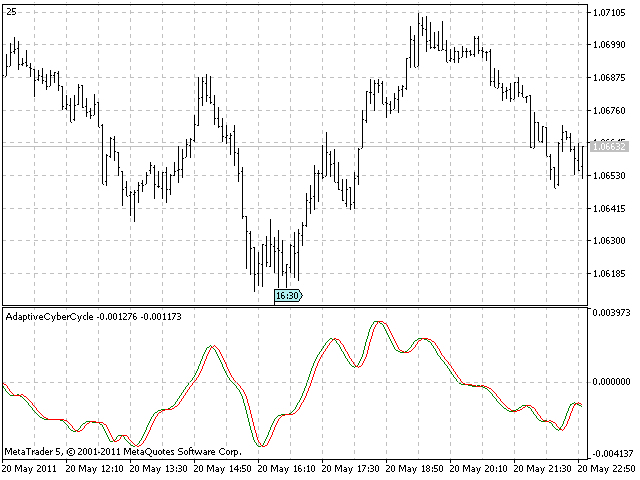Our first adaptive indicator is ready. According to the book it should be more responsive than the non-adaptive version.

Buy and sell signals should often occur one bar earlier than for the non-adaptive version.

We can proceed with two more indicator examples, it should be enough for you to figure out the scheme for creating adaptive indicators.

### Center of Gravity indicator

When speaking of the center of gravity for any physical object we mean its balance point. The idea to introduce this concept into trading came from observing of how lags of various filters were related to filter's coefficients.

For SMA - Simple Moving Average, all coeficients are equal, center of gravity is in the middle.

For WMA - Weighted Moving Average, latest prices are more important than the older ones. To be specific, coefficients of WMA describe outline of the triangle. Triangle's center of gravity is in one-third of the length of the base of the triangle. The more generic equation derived for computing center of gravity on a given observation window is as follows: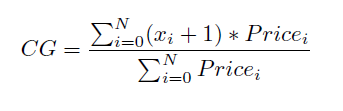The position of the balance point is the summation of the product of position within the window times the price at this position (+1 in the equation was introduced because we count from 0 to N and not from 1 to N) divided by the summation of prices within the window.

The main characteristic of the CG is that is decreases and increases along with price swings and essentially it is a zero-lag oscillator.

Please find the source code below:

```//+------------------------------------------------------------------+
//|                                              CenterOfGravity.mq5 |
//|                                      Copyright 2011, Investeo.pl |
//|                                               http://Investeo.pl |
//+------------------------------------------------------------------+
#property copyright "Copyright 2011, Investeo.pl"
#property link      "http://Investeo.pl"
#property version   "1.00"
#property indicator_separate_window

#property description "CG indicator - described by John F. Ehlers"
#property description "in \"Cybernetic Analysis for Stocks and Futures\""
#property description "This indicator is available for free download."

#property indicator_buffers 2
#property indicator_plots 2
#property indicator_width1 1
#property indicator_width2 1
#property indicator_type1   DRAW_LINE
#property indicator_type2   DRAW_LINE
#property indicator_color1  Green
#property indicator_color2  Red
#property indicator_label1  "Cycle"
#property indicator_label2  "Trigger Line"

#define Price(i) ((high[i]+low[i])/2.0)

double Smooth[];
double Cycle[];
double Trigger[];

input double InpAlpha=0.07; // alpha
input int InpCGLength=10; //CG window size

//+------------------------------------------------------------------+
//| Custom indicator initialization function                         |
//+------------------------------------------------------------------+
int OnInit()
{
//--- indicator buffers mapping
ArraySetAsSeries(Cycle,true);
ArraySetAsSeries(Trigger,true);
ArraySetAsSeries(Smooth,true);

SetIndexBuffer(0,Cycle,INDICATOR_DATA);
SetIndexBuffer(1,Trigger,INDICATOR_DATA);

PlotIndexSetDouble(0,PLOT_EMPTY_VALUE,0.0);
PlotIndexSetDouble(1,PLOT_EMPTY_VALUE,0.0);

return(0);
}
//+------------------------------------------------------------------+
//| Custom indicator iteration function                              |
//+------------------------------------------------------------------+
int OnCalculate(const int rates_total,
const int prev_calculated,
const datetime &time[],
const double &open[],
const double &high[],
const double &low[],
const double &close[],
const long &tick_volume[],
const long &volume[],
const int &spread[])
{
//---
long tickCnt;
int i;
double Num, Denom; // Numerator and Denominator for CG
int ticks=CopyTickVolume(Symbol(), 0, 0, 1, tickCnt);
if(ticks!=1) return(rates_total);

Comment(tickCnt);

if(prev_calculated==0 || tickCnt==1)
{
//--- last counted bar will be recounted
int nLimit=rates_total-prev_calculated-1; // start index for calculations

ArraySetAsSeries(high,true);
ArraySetAsSeries(low,true);

ArrayResize(Smooth,Bars(_Symbol,_Period));
ArrayResize(Cycle,Bars(_Symbol,_Period));

if(nLimit>rates_total-InpCGLength) // adjust for last bars
nLimit=rates_total-InpCGLength;

for(i=nLimit;i>=0 && !IsStopped();i--)
{
Num = 0.0;
Denom = 0.0;
for (int count=0; count<InpCGLength; count++)
{
Num += (1.0+count)*Price(i+count);
Denom += Price(i+count);
}
if (Denom != 0.0)
Cycle[i] = -Num/Denom+(InpCGLength+1.0)/2.0;
else
Cycle[i] = 0.0;

//Print(__FILE__+__FUNCTION__+" received values: ",rCnt);
Trigger[i]=Cycle[i+1];
}
}
//--- return value of prev_calculated for next call
return(rates_total);
}
//+------------------------------------------------------------------+ ```

A screenshot below. Please notice the small lag.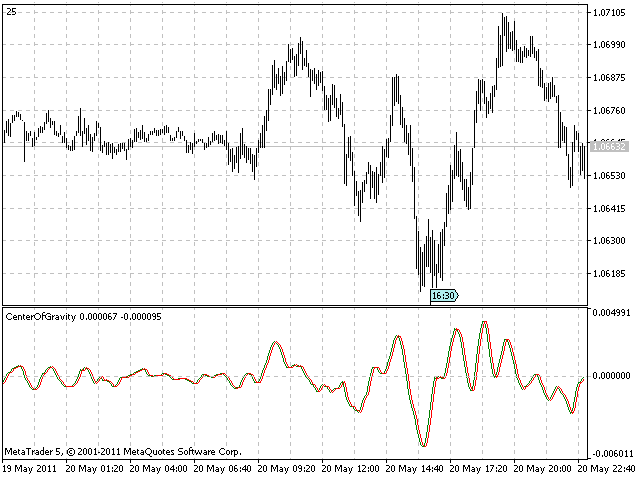### Adaptive Center of Gravity indicator

CG oscillator finds center of gravity on a fixed length time window. The Adaptive CG oscillator uses half the measured Dominant Cycle period as the dynamic window length. In order to extract half the measured Dominant Cycle period the following code was used.

```copied=CopyBuffer(hCyclePeriod,0,i,1,CyclePeriod);

if(copied<=0)
{
Print("FAILURE: Could not get values from CyclePeriod indicator.");
return -1;
}
CG_len = floor(CyclePeriod/2.0);```

Please find full indicator source code below and compare it to its non-adaptive version as well as to Adaptive Cyber Cycle indicator for similarities.

```//+------------------------------------------------------------------+
//|                                      AdaptiveCenterOfGravity.mq5 |
//|                                      Copyright 2011, Investeo.pl |
//|                                               http://Investeo.pl |
//+------------------------------------------------------------------+
#property copyright "Copyright 2011, Investeo.pl"
#property link      "http://Investeo.pl"
#property version   "1.00"
#property indicator_separate_window

#property description "Adaptive CG indicator - described by John F. Ehlers"
#property description "in \"Cybernetic Analysis for Stocks and Futures\""
#property description "This indicator is available for free download."

#property indicator_buffers 2
#property indicator_plots 2
#property indicator_width1 1
#property indicator_width2 1
#property indicator_type1   DRAW_LINE
#property indicator_type2   DRAW_LINE
#property indicator_color1  Green
#property indicator_color2  Red
#property indicator_label1  "Cycle"
#property indicator_label2  "Trigger Line"

#define Price(i) ((high[i]+low[i])/2.0)

double Smooth[];
double Cycle[];
double Trigger[];

int hCyclePeriod;

input double InpAlpha=0.07; // alpha
//+------------------------------------------------------------------+
//| Custom indicator initialization function                         |
//+------------------------------------------------------------------+
int OnInit()
{
//--- indicator buffers mapping
ArraySetAsSeries(Cycle,true);
ArraySetAsSeries(Trigger,true);
ArraySetAsSeries(Smooth,true);

SetIndexBuffer(0,Cycle,INDICATOR_DATA);
SetIndexBuffer(1,Trigger,INDICATOR_DATA);

PlotIndexSetDouble(0,PLOT_EMPTY_VALUE,0.0);
PlotIndexSetDouble(1,PLOT_EMPTY_VALUE,0.0);

hCyclePeriod=iCustom(NULL,0,"CyclePeriod",InpAlpha);
if(hCyclePeriod==INVALID_HANDLE)
{
Print("CyclePeriod indicator not available!");
return(-1);
}

return(0);
}
//+------------------------------------------------------------------+
//| Custom indicator iteration function                              |
//+------------------------------------------------------------------+
int OnCalculate(const int rates_total,
const int prev_calculated,
const datetime &time[],
const double &open[],
const double &high[],
const double &low[],
const double &close[],
const long &tick_volume[],
const long &volume[],
const int &spread[])
{
//---
long tickCnt;
int i, copied;
double Num,Denom; // Numerator and Denominator for CG
double CG_len;
int ticks=CopyTickVolume(Symbol(), 0, 0, 1, tickCnt);
if(ticks!=1) return(rates_total);
double CyclePeriod;

Comment(tickCnt);

if(prev_calculated==0 || tickCnt==1)
{
//--- last counted bar will be recounted
int nLimit=rates_total-prev_calculated-1; // start index for calculations

ArraySetAsSeries(high,true);
ArraySetAsSeries(low,true);

ArrayResize(Smooth,Bars(_Symbol,_Period));
ArrayResize(Cycle,Bars(_Symbol,_Period));

copied=CopyBuffer(hCyclePeriod,0,0,1,CyclePeriod);

if(copied<=0)
{
Print("FAILURE: Could not get values from CyclePeriod indicator.");
return -1;
}

if(nLimit>rates_total-int(CyclePeriod)-2) // adjust for last bars
nLimit=rates_total-int(CyclePeriod)-2;

for(i=nLimit;i>=0 && !IsStopped();i--)
{
copied=CopyBuffer(hCyclePeriod,0,i,1,CyclePeriod);

if(copied<=0)
{
Print("FAILURE: Could not get values from CyclePeriod indicator.");
return -1;
}
CG_len = floor(CyclePeriod/2.0);
//Print("CG_len="+DoubleToString(CG_len));

Num=0.0;
Denom=0.0;
for(int count=0; count<int(CG_len); count++)
{
Num+=(1.0+count)*Price(i+count);
Denom+=Price(i+count);
}
if(Denom!=0.0)
Cycle[i]=-Num/Denom+(CG_len+1.0)/2.0;
else
Cycle[i]=0.0;

//Print(__FILE__+__FUNCTION__+" received values: ",rCnt);
Trigger[i]=Cycle[i+1];
}
}
//--- return value of prev_calculated for next call
return(rates_total);
}
//+------------------------------------------------------------------+```

Please observe the AdaptiveCG indicator screenshot pasted below.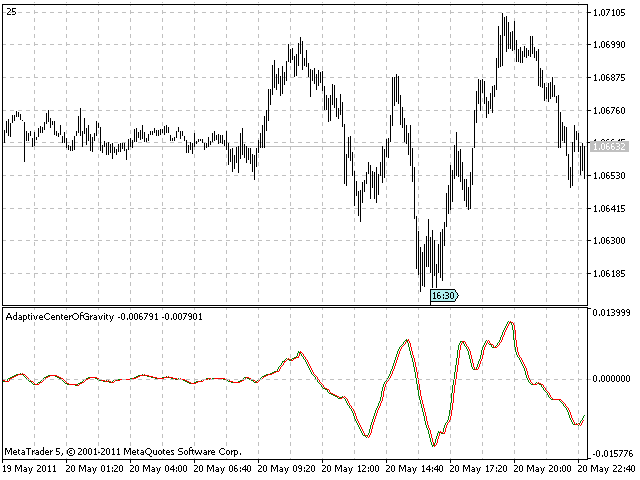### RVI indicator

RVI stands for Relative Vigor Index. The basic theory behind this indicator is that prices tend to have close price higher than open price in bull markets and close price lower than open price in bear markets.

The vigor of the move is measured by the difference of close price to open price relative to daily trading range.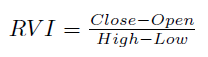This is a quite known indicator for many MetaTrader users as it is now embedded into MetaTrader 5 installation.

I am still pasting the source code for reference:

```//+------------------------------------------------------------------+
//|                                                          RVI.mq5 |
//|                        Copyright 2009, MetaQuotes Software Corp. |
//|                                              https://www.mql5.com |
//+------------------------------------------------------------------+
#property copyright   "2009, MetaQuotes Software Corp."
#property link        "https://www.mql5.com"
#property description "Relative Vigor Index"
//--- indicator settings
#property indicator_separate_window
#property indicator_buffers 2
#property indicator_plots   2
#property indicator_type1   DRAW_LINE
#property indicator_type2   DRAW_LINE
#property indicator_color1  Green
#property indicator_color2  Red
#property indicator_label1  "RVI"
#property indicator_label2  "Signal"
//--- input parameters
input int InpRVIPeriod=10; // Period
//--- indicator buffers
double    ExtRVIBuffer[];
double    ExtSignalBuffer[];
//---
#define TRIANGLE_PERIOD  3
#define AVERAGE_PERIOD   (TRIANGLE_PERIOD*2)
//+------------------------------------------------------------------+
//| Custom indicator initialization function                         |
//+------------------------------------------------------------------+
void OnInit()
{
//--- indicator buffers mapping
SetIndexBuffer(0,ExtRVIBuffer,INDICATOR_DATA);
SetIndexBuffer(1,ExtSignalBuffer,INDICATOR_DATA);
IndicatorSetInteger(INDICATOR_DIGITS,3);
//--- sets first bar from what index will be drawn
PlotIndexSetInteger(0,PLOT_DRAW_BEGIN,(InpRVIPeriod-1)+TRIANGLE_PERIOD);
PlotIndexSetInteger(1,PLOT_DRAW_BEGIN,(InpRVIPeriod-1)+AVERAGE_PERIOD);
//--- name for DataWindow and indicator subwindow label
IndicatorSetString(INDICATOR_SHORTNAME,"RVI("+string(InpRVIPeriod)+")");
PlotIndexSetString(0,PLOT_LABEL,"RVI("+string(InpRVIPeriod)+")");
PlotIndexSetString(1,PLOT_LABEL,"Signal("+string(InpRVIPeriod)+")");
//--- initialization done
}
//+------------------------------------------------------------------+
//| Relative Vigor Index                                             |
//+------------------------------------------------------------------+
int OnCalculate(const int rates_total,const int prev_calculated,
const datetime &Time[],
const double &Open[],
const double &High[],
const double &Low[],
const double &Close[],
const long &TickVolume[],
const long &Volume[],
const int &Spread[])
{
int    i,j,nLimit;
double dValueUp,dValueDown,dNum,dDeNum;
//--- check for bars count
if(rates_total<=InpRVIPeriod+AVERAGE_PERIOD+2) return(0); // exit with zero result
//--- check for possible errors
if(prev_calculated<0) return(0); // exit with zero result
//--- last counted bar will be recounted
nLimit=InpRVIPeriod+2;
if(prev_calculated>InpRVIPeriod+TRIANGLE_PERIOD+2)
nLimit=prev_calculated-1;
//--- set empty value for uncalculated bars
if(prev_calculated==0)
{
for(i=0;i<InpRVIPeriod+TRIANGLE_PERIOD;i++) ExtRVIBuffer[i]=0.0;
for(i=0;i<InpRVIPeriod+AVERAGE_PERIOD;i++)  ExtSignalBuffer[i]=0.0;
}
//--- RVI counted in the 1-st buffer
for(i=nLimit;i<rates_total && !IsStopped();i++)
{
dNum=0.0;
dDeNum=0.0;
for(j=i;j>i-InpRVIPeriod;j--)
{
dValueUp=Close[j]-Open[j]+2*(Close[j-1]-Open[j-1])+2*(Close[j-2]-Open[j-2])+Close[j-3]-Open[j-3];
dValueDown=High[j]-Low[j]+2*(High[j-1]-Low[j-1])+2*(High[j-2]-Low[j-2])+High[j-3]-Low[j-3];
dNum+=dValueUp;
dDeNum+=dValueDown;
}
if(dDeNum!=0.0)
ExtRVIBuffer[i]=dNum/dDeNum;
else
ExtRVIBuffer[i]=dNum;
}
//--- signal line counted in the 2-nd buffer
nLimit=InpRVIPeriod+TRIANGLE_PERIOD+2;
if(prev_calculated>InpRVIPeriod+AVERAGE_PERIOD+2)
nLimit=prev_calculated-1;
for(i=nLimit;i<rates_total && !IsStopped();i++)
ExtSignalBuffer[i]=(ExtRVIBuffer[i]+2*ExtRVIBuffer[i-1]+2*ExtRVIBuffer[i-2]+ExtRVIBuffer[i-3])/AVERAGE_PERIOD;

//--- OnCalculate done. Return new prev_calculated.
return(rates_total);
}
//+------------------------------------------------------------------+
```

A screenshot of the standard RVI indicator with period set to default 10 is pasted below.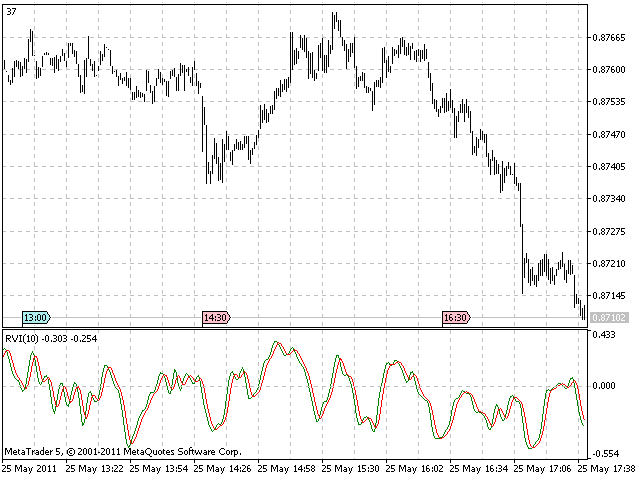### Adaptive RVI indicator

As with the previous two adaptive indicators we need to extract Dominant Cycle measurement from CyclePeriod indicator and apply it to RVI period. The "Length" variable is computed as a four bar weighted moving average of the period:

```copied=CopyBuffer(hCyclePeriod,0,0,4,CyclePeriod);

if(copied<=0)
{
Print("FAILURE: Could not get values from CyclePeriod indicator.");
return -1;
}
AdaptiveRVIPeriod = int(floor((4*CyclePeriod+3*CyclePeriod+2*CyclePeriod+CyclePeriod)/20.0));```

Please find the full source code of the Adaptive RVI indicator below.

```//+------------------------------------------------------------------+
//|                                                 Adaptive RVI.mq5 |
//|                        Based on RVI by MetaQuotes Software Corp. |
//|                        Copyright 2009, MetaQuotes Software Corp. |
//|                                              https://www.mql5.com |
//+------------------------------------------------------------------+
#property copyright   "2009, MetaQuotes Software Corp."
#property copyright   "2011, Adaptive version Investeo.pl"
#property link        "https://www.mql5.com"
#property description "Adaptive Relative Vigor Index"
//--- indicator settings
#property indicator_separate_window
#property indicator_buffers 2
#property indicator_plots   2
#property indicator_type1   DRAW_LINE
#property indicator_type2   DRAW_LINE
#property indicator_color1  Green
#property indicator_color2  Red
#property indicator_label1  "AdaptiveRVI"
#property indicator_label2  "Signal"

#define Price(i) ((high[i]+low[i])/2.0)

//--- input parameters
input int InpRVIPeriod=10; // Initial RVI Period
//--- indicator buffers
double    ExtRVIBuffer[];
double    ExtSignalBuffer[];
//---
int hCyclePeriod;
input double InpAlpha=0.07; // alpha for Cycle Period
int AdaptiveRVIPeriod;

#define TRIANGLE_PERIOD  3
#define AVERAGE_PERIOD   (TRIANGLE_PERIOD*2)
//+------------------------------------------------------------------+
//| Custom indicator initialization function                         |
//+------------------------------------------------------------------+
int OnInit()
{
//--- indicator buffers mapping
SetIndexBuffer(0,ExtRVIBuffer,INDICATOR_DATA);
SetIndexBuffer(1,ExtSignalBuffer,INDICATOR_DATA);
IndicatorSetInteger(INDICATOR_DIGITS,3);
hCyclePeriod=iCustom(NULL,0,"CyclePeriod",InpAlpha);
if(hCyclePeriod==INVALID_HANDLE)
{
Print("CyclePeriod indicator not available!");
return(-1);
}

//--- sets first bar from what index will be drawn
PlotIndexSetInteger(0,PLOT_DRAW_BEGIN,(InpRVIPeriod-1)+TRIANGLE_PERIOD);
PlotIndexSetInteger(1,PLOT_DRAW_BEGIN,(InpRVIPeriod-1)+AVERAGE_PERIOD);
//--- name for DataWindow and indicator subwindow label
IndicatorSetString(INDICATOR_SHORTNAME,"AdaptiveRVI");
PlotIndexSetString(0,PLOT_LABEL,"AdaptiveRVI");
PlotIndexSetString(1,PLOT_LABEL,"Signal");
//--- initialization done
return 0;
}
//+------------------------------------------------------------------+
//| Relative Vigor Index                                             |
//+------------------------------------------------------------------+
int OnCalculate(const int rates_total,const int prev_calculated,
const datetime &Time[],
const double &Open[],
const double &High[],
const double &Low[],
const double &Close[],
const long &TickVolume[],
const long &Volume[],
const int &Spread[])
{
int    i,j,nLimit;
double dValueUp,dValueDown,dNum,dDeNum;
double CyclePeriod;
int copied;

copied=CopyBuffer(hCyclePeriod,0,0,4,CyclePeriod);

if(copied<=0)
{
Print("FAILURE: Could not get values from CyclePeriod indicator.");
return -1;
}
AdaptiveRVIPeriod = int(floor((4*CyclePeriod+3*CyclePeriod+2*CyclePeriod+CyclePeriod)/20.0));
//--- check for bars count
if(rates_total<=AdaptiveRVIPeriod+AVERAGE_PERIOD+2) return(0); // exit with zero result
//--- check for possible errors
if(prev_calculated<0) return(0); // exit with zero result
//--- last counted bar will be recounted
nLimit=AdaptiveRVIPeriod+2;
if(prev_calculated>AdaptiveRVIPeriod+TRIANGLE_PERIOD+2)
nLimit=prev_calculated-1;
//--- set empty value for uncalculated bars
if(prev_calculated==0)
{
for(i=0;i<AdaptiveRVIPeriod+TRIANGLE_PERIOD;i++) ExtRVIBuffer[i]=0.0;
for(i=0;i<AdaptiveRVIPeriod+AVERAGE_PERIOD;i++)  ExtSignalBuffer[i]=0.0;
}
//--- RVI counted in the 1-st buffer
for(i=nLimit;i<rates_total && !IsStopped();i++)
{
copied=CopyBuffer(hCyclePeriod,0,rates_total-i-1,4,CyclePeriod);

if(copied<=0)
{
Print("FAILURE: Could not get values from CyclePeriod indicator.");
return -1;
}
AdaptiveRVIPeriod = int(floor((4*CyclePeriod+3*CyclePeriod+2*CyclePeriod+CyclePeriod)/20.0));
dNum=0.0;
dDeNum=0.0;
for(j=i;j>MathMax(i-AdaptiveRVIPeriod, 3);j--)
{
//Print("rates_total="+IntegerToString(rates_total)+" nLimit="+IntegerToString(nLimit)+
//      " AdaptiveRVIPeriod="+IntegerToString(AdaptiveRVIPeriod)+" j="+IntegerToString(j));
dValueUp=Close[j]-Open[j]+2*(Close[j-1]-Open[j-1])+2*(Close[j-2]-Open[j-2])+Close[j-3]-Open[j-3];
dValueDown=High[j]-Low[j]+2*(High[j-1]-Low[j-1])+2*(High[j-2]-Low[j-2])+High[j-3]-Low[j-3];
dNum+=dValueUp;
dDeNum+=dValueDown;
}
if(dDeNum!=0.0)
ExtRVIBuffer[i]=dNum/dDeNum;
else
ExtRVIBuffer[i]=dNum;
}
//--- signal line counted in the 2-nd buffer
nLimit=AdaptiveRVIPeriod+TRIANGLE_PERIOD+2;
if(prev_calculated>AdaptiveRVIPeriod+AVERAGE_PERIOD+2)
nLimit=prev_calculated-1;
for(i=nLimit;i<rates_total && !IsStopped();i++)
ExtSignalBuffer[i]=(ExtRVIBuffer[i]+2*ExtRVIBuffer[i-1]+2*ExtRVIBuffer[i-2]+ExtRVIBuffer[i-3])/AVERAGE_PERIOD;

//--- OnCalculate done. Return new prev_calculated.
return(rates_total);
}
//+------------------------------------------------------------------+ ```

A screenshot of Adaptive RVI indicator with dynamic window length: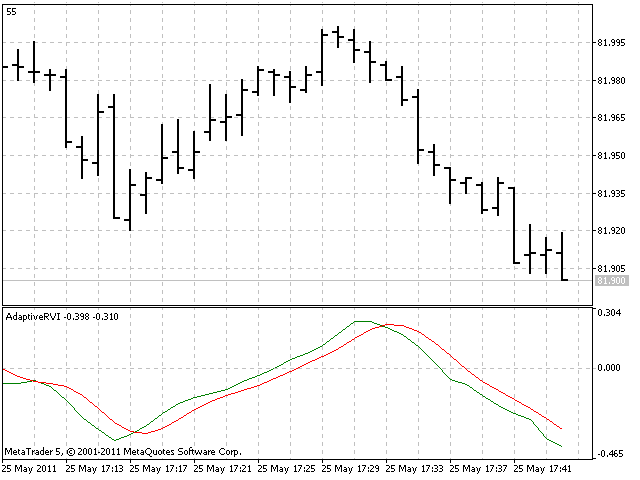### Conclusion

This article presented three adaptive technical indicators behaviour and MQL5 implementation.

The mechanism for implementing the adaptive indicators should be clearly understandable after reading the article. All described indicators are available in attachments.

The author encourages to experiment and build other adaptive indicators from the ones already available.

Attached files |
adaptivervi.mq5 (5.63 KB)
cybercycle.mq5 (4.09 KB)
cycleperiod.mq5 (6.04 KB)

#### Other articles by this author

Last comments | Go to discussion (9)| 16 Aug 2011 at 19:36
CyclePeriod.mq5 is located in ./MQL5/Indicators.
No compilation errors or warnings of the file.
When I am looking for CyclePeriod.ex5 file, I can not find it on my local disk ?| 16 Aug 2011 at 19:43
I find the issue=> it is OK now| 22 May 2013 at 12:27

The indicators should be one of the best indicators written in MQL5. As a beginner  for C++ and newcomer for MQL5, some of your codes give difficulty to me when I replicate it into C++.. For example C++ doesn't have isStopped()..

Is there any easier way of porting your code into C++?, specially code for adaptive cycle function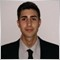| 1 Mar 2016 at 20:14

Someone knows how to change the interval period inded of 6 to 63 how the article says?

Thanks, in advance.| 1 Mar 2016 at 22:01

I find out the way to change the variable period for another different changing the costant 6.28318 and maybe the compesation number 0.5 at this equation "DC =(6.28318/MedianDelta)+0.5;" at the code of cycle period, if someone has the same doubt.

I've realized that maybe there is a mistake in the article or It's not very clear why describes DeltaPhase is restricted between <0.1, 1.1> and in the code it's between <0.1, 0.9>.

I want to say that this is only my doubts and my thinkings but it's a great article.

Thanks a lot.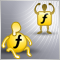Decreasing Memory Consumption by Auxiliary Indicators
If an indicator uses values of many other indicators for its calculations, it consumes a lot of memory. The article describes several methods of decreasing the memory consumption when using auxiliary indicators. Saved memory allows increasing the number of simultaneously used currency pairs, indicators and strategies in the client terminal. It increases the reliability of trade portfolio. Such a simple care about technical resources of your computer can turn into money resources at your deposit.The Fundamentals of Testing in MetaTrader 5
What are the differences between the three modes of testing in MetaTrader 5, and what should be particularly looked for? How does the testing of an EA, trading simultaneously on multiple instruments, take place? When and how are the indicator values calculated during testing, and how are the events handled? How to synchronize the bars from different instruments during testing in an "open prices only" mode? This article aims to provide answers to these and many other questions.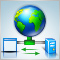Using WinInet in MQL5. Part 2: POST Requests and Files
In this article, we continue studying the principles of working with Internet using HTTP requests and exchange of information with server. It describes new functions of the CMqlNet class, methods of sending information from forms and sending of files using POST requests as well as authorization on websites under your login using Cookies.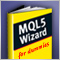MQL5 Wizard for Dummies
In early 2011 we released the first version of the MQL5 Wizard. This new application provides a simple and convenient tool to automatically generate trading robots. Any MetaTrader 5 user can create a custom Expert Advisor without even knowing how to program in MQL5.